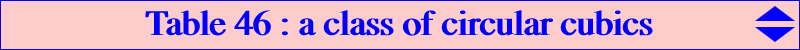This page is devoted to a class of cubics which solve a problem raised by Dominik Burek (ADGEOM # 579) and partially answered by Francisco Garcia Capitan See the related paper Cubics related to concurrent tangents *** Consider a fixed point P = p : q : r and a variable point Q. The circumcircle of APQ meets AB, AC again at Ab, Ac. Define Bc, Ba and Ca, Cb likewise. These six points lie on a same conic if and only if Q lies on a circular circum-cubic denoted by K(P) with equation : ∑ a^4 q r y z [p (y- z) - (q -r )x] + b^2 c^2 p x [r^2 y (x+z) - q^2 z (x+y) + r (p+q) y^2 - q (p+r) z^2] = 0. In ADGEOM #612, we find another characterization of K(P) which is the locus of Q such that the tangents at A, B, C to the circles APQ, BPQ, CPQ concur (at X). In this case, the locus of X is another circular circum-cubic X(P) passing through X(3) with equation : ∑ p^2 (r y-q z) [b^2 c^2 x^2 - 2 a^2 SA y z + c^2(a^2 - c^2) x y + b^2(a^2 - b^2) x z] = 0. *** General properties of K(P) K(P) contains : • A, B, C, the circular points at infinity J1 and J2, P and its isogonal conjugate gP = P*, • the infinite point of the line cP-mP where cP = complement of P and mP = midpoint(P, P*), • the isogonal conjugate of the infinite point of the line cgP-mP, this point obviously on the circumcircle. K(P*) is the isogonal transform of K(P) and these two cubics generate a pencil of circular circum-cubics passing through P, P* that also contains : • the isogonal pK with pivot the infinite point of the line PP*, • the isogonal focal nK whose root is the trilinear pole of the last common tangent of the Steiner inellipse and the inscribed conic with foci P and P*, with center mP. All these cubics must meet at two other (real or not) isogonal conjugate points P1, P2 lying on a parallel to PP*. *** Special cubics K(P) • K(O) splits into the line at infinity and the Jerabek hyperbola. • K(H) = K(O)* splits into the circumcircle and the Euler line. • K(P) is a focal cubic if and only if P lies on the Napoleon cubic K005. • K(P) is a pK if and only if P lies on the second Brocard cubic K018. In this case, the pivot lies on the Kiepert hyperbola, the isopivot lies on the Brocard axis, the pole lies on the cubic K222. • K(P) is a nK if and only if P lies on a complicated tricircular isogonal sextic passing through the in/excenters, O, H. • K(P) is a psK if and only if P lies on the line at infinity (1) or on the circumcircle (2). More precisely, - (1) K(P) = psK(P x X6, X2, X3) contains O, the midpoints of ABC. The singular focus lies on C(O, R/2) and the pseudo-pole lies on the Lemoine axis. - (2) K(P) = psK(P, P x X76, X4) contains H and is tangent at A, B, C to the symmedians. The singular focus lies on C(O, 2R) and the pseudo-pivot lies on the Steiner ellipse. In this case, K(P) is the locus of the pivots of circular pKs passing through P. *** General properties of X(P) X(P) contains : • A, B, C, the circular points at infinity J1 and J2, O, P, igP = inverse in the circumcircle of gP, • the infinite point of the line P-H/P where H/P is the H-Ceva conjugate of P, • the isogonal conjugate of the infinite point of the line P-gP, this point obviously on the circumcircle. *** Special cubics X(P) • X(H) splits into the line at infinity and the Jerabek hyperbola. • X(I) splits into the circumcircle and the line OI. • X(P) is a focal cubic if and only if P lies on the Napoleon cubic K005. • X(P) is a pK if and only if P lies on the second Brocard cubic K018. • the polar conic of O is a rectangular hyperbola if and only if P lies on the Neuberg cubic K001. • when P lies on the line at infinity, the cubics X(P) and K(P) coincide. *** The following table gives a selection of such cubics K(P)P cubic K(P) or centers --- cubic X(P) type remarks about K(P) X(1) K086 --- (O) +line OI focal cK a strophoid X(2) K273 --- K043 pK DF cubic X(5) K464 --- K465 focal X(6) K043 --- K108 pK X(13) K261a --- K001 pK orthopivotal cubics X(14) K261b --- K001 pK X(15) K261a --- K073 pK X(16) K261b --- K073 pK X(30) K446 psK X(54) K467 focal X(74) K447 psK X(106) X(1), X(4), X(106), X(519), X(1168), X(1320) psK X(110) K568 psK X(111) K273 --- K108 pK DF cubic X(511) K570 psK X(523) K567 psK X(524) K043 psK X(759) K306 psK ? K336 pK inversible cubic ? K337 pK antigonal cubic ? K653 pK X(1141) X(4), X(54), X(265), X(1141), X(1154) psK X(2291) X(4), X(9), X(57), X(527), X(1156), X(2291) psK X(2373) X(4), X(69), X(316), X(1177), X(2373), X(2393) psK X(2380) X(4), X(14), X(15), X(532), X(1337), X(2380) psK X(2381) X(4), X(13), X(16), X(533), X(1338), X(2381) psK